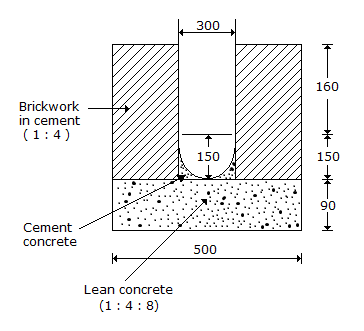# Civil Engineering - Estimating and Costing - Discussion

### Discussion :: Estimating and Costing - Section 2 (Q.No.37)

37.

The cost of the earthwork in excavation for the surface drain of cross-section shown in the given figure for a total length of 5 metres @ Rs. 450% cum, is[A]. Rs. 400 [B]. Rs. 425 [C]. Rs. 450 [D]. Rs. 500

Explanation:

No answer description available for this question.

 Rashad said: (Sep 1, 2015) Size = 0.4*0.05 = 0.2. Length = 5 meters. = 0.2*5*450 = 450. C is the right answer.

 Kiran Poola said: (Oct 31, 2015) 0.4*0.5 = 0.2. L = 5 m = 5*0.2*450 = 450.

 Rajnish said: (Jan 28, 2016) It ask for cutting not total.

 Ranjitha said: (May 4, 2016) @Rajnish The length of drain = 5m. Width of drain as per figure = 0.5m. Depth of drain as per figure = 0.4m. Total qty = 5 * 0.5 * 0.4 = 1 cum. As per the question, cum excavation rate is 450%. So the total cost required for excavation of drain is = 1 * 450 = Rs.450. Therefore, the answer is C.

 Usha said: (Nov 19, 2016) @Ranjitha is correct.

 M.Rashid said: (Feb 4, 2017) C is the right answer. I agree.

 Pratap said: (Feb 9, 2017) I think that Rs. 450 is given for per 100 cum. Which means its Rs. 4.5 per cum.

 Rahul Kushwaha said: (Apr 7, 2017) 5*0.5*0.4=1cum at 450 = Rs.450 is right. So the answer is C.

 Notin said: (May 18, 2017) Out of the quantities of various item earthwork in an excavation in the foundation, PCC works in the foundation with 1:4:8 proportion, bricks work 1:6 foundation and plinth, damp proof course 2.5 cm thick, plastering 1.6 both side of wall and plinth wall.

 Sitaam said: (Dec 1, 2017) 5*.5 *.4 = 1 * 450 = 450 answer.

 Ranguwal said: (Mar 29, 2018) The dimensions in figure are in which unit? mm, m, cm? Please tell me.

 Ranguwal said: (Mar 29, 2018) According to the answer, the dimensions should be in cm. So, Quantity is - (500 cm ) x (400 cm ) x (500 cm) or 5m x 4m x 5m = 20m x 5m =100m & rate is 450 for %cum means 450 rs for 100cum and answer is 450.

 Saurabh Garg said: (Oct 17, 2018) E/W= 5 * 0.5 * 0.4 = 1 m3. The Cost of E/W= 1 * 450 = 450.